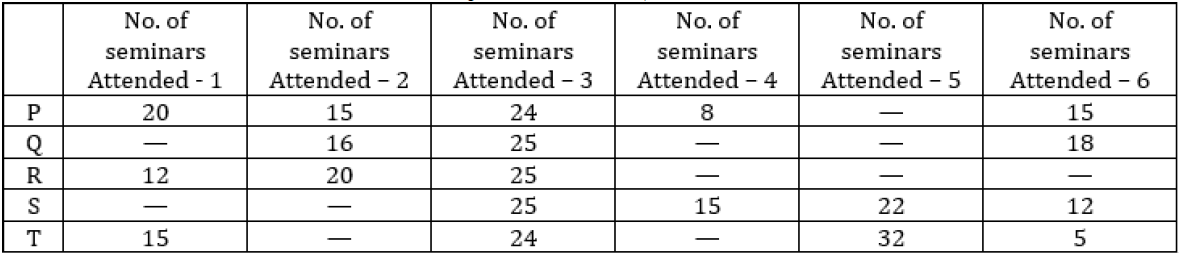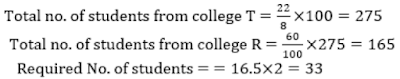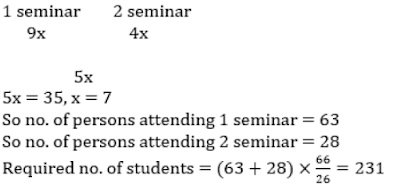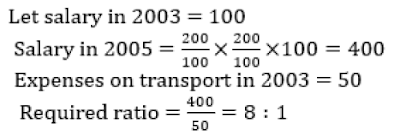# Data Interpretation for SBI PO 2017

Directions (1 – 5): Given below is the percentage distribution of number of students from 5 different colleges attended different number of seminars.1. If the number of students from college R who attended at most 3 seminars is equal to number of students from college S who attended at least 3 seminars and the total number of students from College S is 114. Then find the number of students from college R who attended 3 seminars.
A. 37
B. 41
C. 45
D. 48
E. None of these
2. Total no. of students from college P who attended at-most 2 seminars is equal to the sum of number of students from college T who attended 1 seminar and the number of students from the same college who attended 6 seminars. Then the total number of students from college P is what percent of total no. of student from college T?
A. 57 1/7%
B. 45%
C. 49%
D. 60%
E. None of these
3. The number of students from college P who attended more than 2 seminars is approximate what percent less than the number of students from college S who attended at least 3 seminars if the number of student who attended 2 seminars from college P is 48. And the number of students of college S who attended 6 seminars is 14 more than the number of students from college P, who attended 1 seminar?
A. 57%
B. 44%
C. 60%
D. 48%
E. 52%
4. If the difference between number of students from college T who attended 3 seminars and students who attended 5 seminars is 22, and the total students from college R is 60% of the total students from college T then find the number of students of college R who are attending 2 seminars.
A. 32
B. 35
C. 33
D. 38
E. None of these
5. If the ratio between the no. of students who attended 1 seminar and the no. of students who attend 2 seminars from college S is 9 : 4, and the difference between the same is 35 Then find the persons from this college who attended at most 4 seminars.
A. 237
B. 234
C. 231
D. 240
E. None of these
Directions (6 – 10): The following bar graph shows the percentage break-up of a person’s salary from year 2001 to 2005. With the given information, answer the following questions.6. If the ratio on saving in the year 2002 and 2005 are in the ratio 7 : 5. Then what is the ratio of EMI expenses in the year 2002 and 2005.
A. 6 : 7
B. 8 : 7
C. 8 : 9
D. Can’t be determined
E. None of these
7. If the saving in 2002 is 3/5th of the saving in 2004. Then what is the total expenditure spent on transport in 2002. (Given that total salary in 2004 is Rs. 1,75,000 Rs.)
A. 31100 Rs.
B. 26100 Rs.
C. 21100 Rs.
D. 15100 Rs.
E. None of these
8. In every year there is an increase of 100% in monthly salary as compared to previous year’s monthly salary then what is the ratio of monthly salary in 2005 to the expenses on transport in 2003.
A. 8:1
B. 1:8
C. 4:1
D. 1 : 4
E. None of these
9. In which year the ratio of EMI expanses to saving is 3rd highest?
A. 2001
B. 2002
C. 2003
D. 2004
E. None of these
10. Find the average percentage of transport expanses over the years.
A. 32%
B. 34%
C. 35%
D. 36%
E. None of these
Solutions:
1. A)2. A)3. A)4. C)5. C)6. B)7. B)8. A)9. B) In 2002 EMI Expenses : Savings = 40/42 and it is the third highest
10. A) Required Average = (40 + 18 + 50 + 17 + 35)/5 = 32%